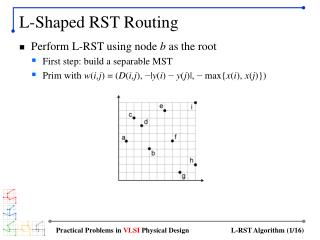DownloadDownload PresentationL-Shaped RST Routing

# L-Shaped RST Routing

Télécharger la présentation## L-Shaped RST Routing

- - - - - - - - - - - - - - - - - - - - - - - - - - - E N D - - - - - - - - - - - - - - - - - - - - - - - - - - -
##### Presentation Transcript

1. L-Shaped RST Routing • Perform L-RST using node b as the root • First step: build a separable MST • Prim with w(i,j) = (D(i,j), −|y(i) −y(j)|, − max{x(i), x(j)}) Practical Problems in VLSI Physical Design

2. First Iteration Practical Problems in VLSI Physical Design

3. Separable MST Construction Practical Problems in VLSI Physical Design

4. Separable MST Construction (cont) Practical Problems in VLSI Physical Design

5. Constructing a Rooted Tree • Node b is the root node • Based on the separable MST (initial wirelength = 32) • Bottom-up traversal is performed on this tree during L-RST routing Practical Problems in VLSI Physical Design

6. Partial L-RST for Node C Practical Problems in VLSI Physical Design

7. Partial L-RST for Node E Practical Problems in VLSI Physical Design

8. Partial L-RST for Node G Practical Problems in VLSI Physical Design

9. Partial L-RST for Node D Practical Problems in VLSI Physical Design

10. Partial L-RST for Node D (cont) Practical Problems in VLSI Physical Design

11. Partial L-RST for Node F best case Practical Problems in VLSI Physical Design

12. Partial L-RST for Node F (cont) best case Practical Problems in VLSI Physical Design

13. Processing the Root Node best case Practical Problems in VLSI Physical Design

14. Top-down Traversal • In order to obtain the final tree upper upper upper lower lower lower upper lower/ upper Practical Problems in VLSI Physical Design

15. Final Tree • Wirelength reduction • Initial wirelength − total overlap = 32 − 4 = 28 Practical Problems in VLSI Physical Design

16. Stable Under Rerouting • Steiner points are marked X • Wirelength does not reduce after rerouting Practical Problems in VLSI Physical Design